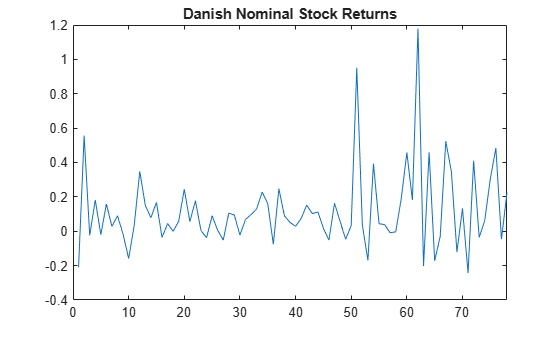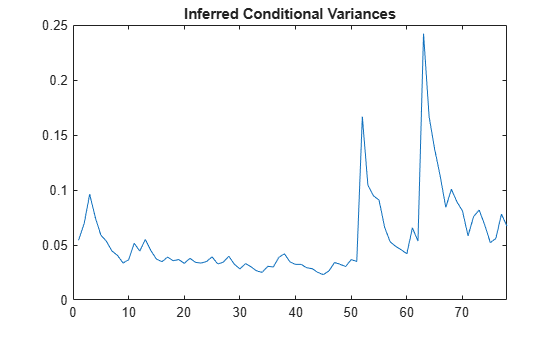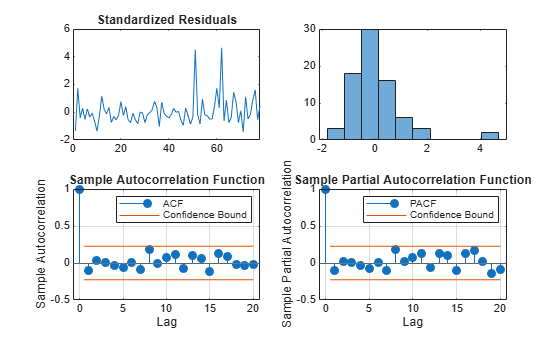# Infer Conditional Variances and Residuals

This example shows how to infer conditional variances from a fitted conditional variance model. Standardized residuals are computed using the inferred conditional variances to check the model fit.

### Step 1. Load the data.

Load the Danish nominal stock return data included with the toolbox.

```load Data_Danish y = DataTable.RN; T = length(y); figure plot(y) xlim([0,T]) title('Danish Nominal Stock Returns')```The return series appears to have a nonzero mean offset and volatility clustering.

### Step 2. Fit an EGARCH(1,1) model.

Specify, and then fit an EGARCH(1,1) model to the nominal stock returns series. Include a mean offset, and assume a Gaussian innovation distribution.

```Mdl = egarch('Offset',NaN','GARCHLags',1,... 'ARCHLags',1,'LeverageLags',1); EstMdl = estimate(Mdl,y);```
``` EGARCH(1,1) Conditional Variance Model with Offset (Gaussian Distribution): Value StandardError TStatistic PValue _________ _____________ __________ _________ Constant -0.62723 0.74401 -0.84304 0.3992 GARCH{1} 0.77419 0.23628 3.2766 0.0010507 ARCH{1} 0.38636 0.37361 1.0341 0.30107 Leverage{1} -0.002499 0.19222 -0.013001 0.98963 Offset 0.10325 0.037727 2.7368 0.0062047 ```

### Step 3. Infer the conditional variances.

Infer the conditional variances using the fitted model.

```v = infer(EstMdl,y); figure plot(v) xlim([0,T]) title('Inferred Conditional Variances')```The inferred conditional variances show increased volatility at the end of the return series.

### Step 4. Compute the standardized residuals.

Compute the standardized residuals for the model fit. Subtract the estimated mean offset, and divide by the square root of the conditional variance process.

```res = (y-EstMdl.Offset)./sqrt(v); figure subplot(2,2,1) plot(res) xlim([0,T]) title('Standardized Residuals') subplot(2,2,2) histogram(res,10) subplot(2,2,3) autocorr(res) subplot(2,2,4) parcorr(res)```The standardized residuals exhibit no residual autocorrelation. There are a few residuals larger than expected for a Gaussian distribution, but the normality assumption is not unreasonable.